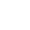﻿

/    2018-09-14

MySQL服务器上进行查询，可以启用高速查询缓存。让数据库引擎在后台悄悄的处理是提高性能的有效方法之一。当同一个查询被执行多次时，如果结果是从缓存中提取，那是相当快的。

```// query cache does NOT work
\$r = mysql_query("SELECT username FROM user WHERE signup_date >=
CURDATE()");
// query cache works!
\$today = date("Y-m-d");
\$r = mysql_query("SELECT username FROM user WHERE signup_date >=
'\$today'");
// query cache does NOT work
\$r = mysql_query("SELECT username FROM user WHERE signup_date >=
CURDATE()");
// query cache works!
\$today = date("Y-m-d");
\$r = mysql_query("SELECT username FROM user WHERE signup_date >=
'\$today'");```

EXPLAIN查询的结果，可以告诉你那些索引正在被引用，表是如何被扫描和排序的等等。```// do I have any users from Alabama?
// what NOT to do:
\$r = mysql_query("SELECT * FROM user WHERE state = 'Alabama'");
if (mysql_num_rows(\$r) > 0) {
// ...
}
// much better:
\$r = mysql_query("SELECT 1 FROM user WHERE state = 'Alabama' LIMIT 1");
if (mysql_num_rows(\$r) > 0) {
// ...
}``````// looking for companies in my state
\$r = mysql_query("SELECT company_name FROM users
LEFT JOIN companies ON (users.state = companies.state)
WHERE users.id = \$user_id");
// both state columns should be indexed
// and they both should be the same type and character encoding
// or MySQL might do full table scans```

```// what NOT to do:
\$r = mysql_query("SELECT username FROM user ORDER BY RAND() LIMIT 1");
// much better:
\$r = mysql_query("SELECT count(*) FROM user");
\$d = mysql_fetch_row(\$r);
\$rand = mt_rand(0,\$d - 1);
\$r = mysql_query("SELECT username FROM user LIMIT \$rand, 1");```

```始终指 定你需要的列，这是一个非常良好的习惯。
// not preferred
\$r = mysql_query("SELECT * FROM user WHERE user_id = 1");
\$d = mysql_fetch_assoc(\$r);
// better:
\$r = mysql_query("SELECT username FROM user WHERE user_id = 1");
\$d = mysql_fetch_assoc(\$r);
// the differences are more significant with bigger result sets```

ROCEDURE ANALYSE()可让MySQL的柱结构分析和表中的实际数据来给你一些建议。如果你的表中已经存在实际数据了，能为你的重大决策服务。```// create a prepared statement
if (\$stmt = \$mysqli->prepare("SELECT username FROM user WHERE state=?"))
{
// bind parameters
\$stmt->bind_param("s", \$state);
// execute
\$stmt->execute();
// bind result variables
// fetch value
\$stmt->fetch();
printf("%s is from %s\n", \$username, \$state);
\$stmt->close();
}```

```\$r = "UPDATE users SET ip = INET_ATON('{\$_SERVER['REMOTE_ADDR']}') WHERE
user_id = \$user_id";```(8)

﻿